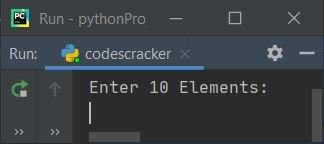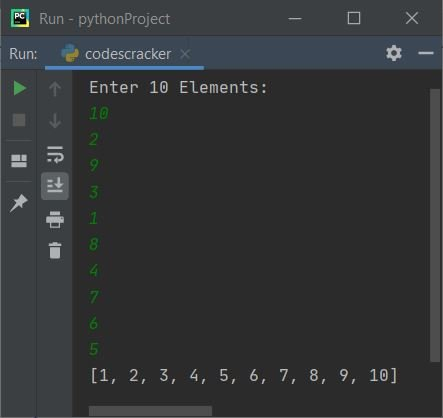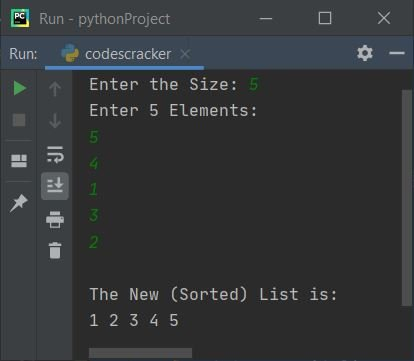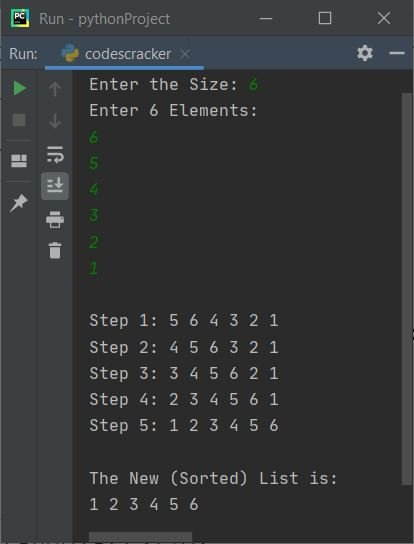# Insertion Sort Program in Python

This article deals with some insertion-sort programs in Python. Insertion sort is a technique used to sort lists in Python. If you're not aware of the topic, then refer to the Insert Sort Algorithm to get all the required items.

Here is a list of insertion sort programs available in this article:

• Insertion sort based on a list of 10 elements entered by the user.
• Insertion sort based on a list of n elements.
• Insertion sort along with the printing of the list after each sort.

## Insertion Sort based on a List of 10 Elements

The question is: write a Python program to sort elements of a list using the insertion sort technique. Here is its answer:

```arr = []
print("Enter 10 Elements: ")
for i in range(10):
arr.append(int(input()))

for i in range(1, 10):
elem = arr[i]
if elem<arr[i-1]:
for j in range(i+1):
if elem<arr[j]:
index = j
for k in range(i, j, -1):
arr[k] = arr[k-1]
break
else:
continue
arr[index] = elem

print(arr)```

Here is the initial output of the above program's sample run:Now supply the input, say 10, 2, 9, 3, 1, 8, 4, 7, 6, 5 as 10 elements to sort the list and print the new list in sorted order:### Allow users to define the size of the list

This program allows the user to define the size of a list along with its elements. The question is: write a Python program to apply insertion sort to a list of n elements. The following program is the answer to this question:

```arr = []
print(end="Enter the Size: ")
arrSize = int(input())
print("Enter " +str(arrSize)+ " Elements: ")
for i in range(arrSize):
arr.append(int(input()))

for i in range(1, arrSize):
elem = arr[i]
if elem<arr[i-1]:
for j in range(i+1):
if elem<arr[j]:
index = j
for k in range(i, j, -1):
arr[k] = arr[k-1]
break
else:
continue
arr[index] = elem

print("\nThe New (Sorted) List is: ")
for i in range(arrSize):
print(end=str(arr[i]) + " ")

print()```

Here is its sample run with user input: 5 as size, 5, 4, 1, 3, 2 as five elements:### Print the list after each insertion sort

This is the last program in this article. The advantage of this program is that you will see a list after each sort operation. Let's have a look at the program and its sample output to understand it in a better way:

```nums = []
print(end="Enter the Size: ")
numsSize = int(input())
print("Enter " +str(numsSize)+ " Elements: ")
for i in range(numsSize):
nums.append(int(input()))

for i in range(1, numsSize):
elem = nums[i]
if elem<nums[i-1]:
for j in range(i+1):
if elem<nums[j]:
index = j
for k in range(i, j, -1):
nums[k] = nums[k-1]
break
else:
continue
nums[index] = elem
print(end="\nStep " +str(i)+ ": ")
for j in range(numsSize):
print(end=str(nums[j]) + " ")

print("\n\nThe New (Sorted) List is: ")
for i in range(numsSize):
print(end=str(nums[i]) + " ")

print()```

Here is its sample run with user input: 6 as size, 6, 5, 4, 3, 2, 1 as six elements:Python Online Test

« Previous Program Next Program »